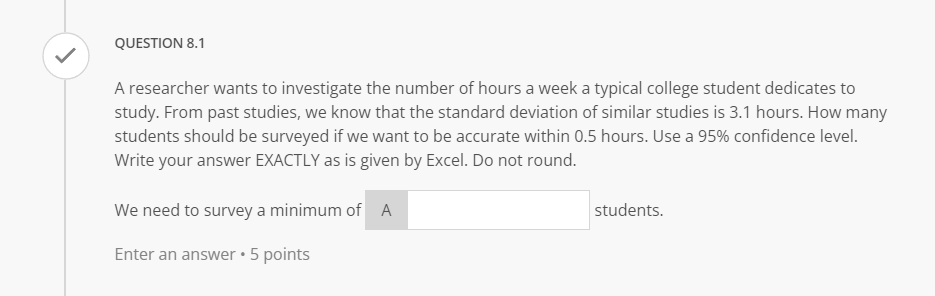# Question Solved1 AnswerQUESTION 8.1 A researcher wants to investigate the number of hours a week a typical college student dedicates to study. From past studies, we know that the standard deviation of similar studies is 3.1 hours. How many students should be surveyed if we want to be accurate within 0.5 hours. Use a 95% confidence level. Write your answer EXACTLY as is given by Excel. Do not round. We need to survey a minimum of A students. Enter an answer. 5 pointsIGFZGO The Asker · Probability and StatisticsTranscribed Image Text: QUESTION 8.1 A researcher wants to investigate the number of hours a week a typical college student dedicates to study. From past studies, we know that the standard deviation of similar studies is 3.1 hours. How many students should be surveyed if we want to be accurate within 0.5 hours. Use a 95% confidence level. Write your answer EXACTLY as is given by Excel. Do not round. We need to survey a minimum of A students. Enter an answer. 5 points
More
Transcribed Image Text: QUESTION 8.1 A researcher wants to investigate the number of hours a week a typical college student dedicates to study. From past studies, we know that the standard deviation of similar studies is 3.1 hours. How many students should be surveyed if we want to be accurate within 0.5 hours. Use a 95% confidence level. Write your answer EXACTLY as is given by Excel. Do not round. We need to survey a minimum of A students. Enter an answer. 5 points